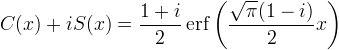Computes the Fresnel cosine and sine integrals.

Namespace:  Meta.Numerics.Functions
Assembly:  Meta.Numerics (in Meta.Numerics.dll) Version: 4.1.4Syntax
```public static Complex Fresnel(
double x
)```

#### Parameters

x
Type: SystemDouble
The argument.

#### Return Value

Type: Complex
The value C(x) + i S(x).Remarks

If you need both C(x) and S(x), it is more efficient to call this function than to call FresnelC(Double) and FresnelS(Double) seperately.

Our definition of C(x) and S(x) agrees with that of Abromowitz and Stegun, the NIST Library of Mathematical Functions, Numerical Recipies, Mathematica, Matlab, SciPy, and any every other reference book and numerical software library I can find. Wikipedia, however uses an alternative definition.

A plot of all values of this function in the complex plane as x ranges from negative infinity to positive infinity is called a Cornu spiral.

The Fresnel function can be related to the complex error function along the line (1-𝑖).See Also